## Easy Development Kit（EDK）的基本使用方法

### 前言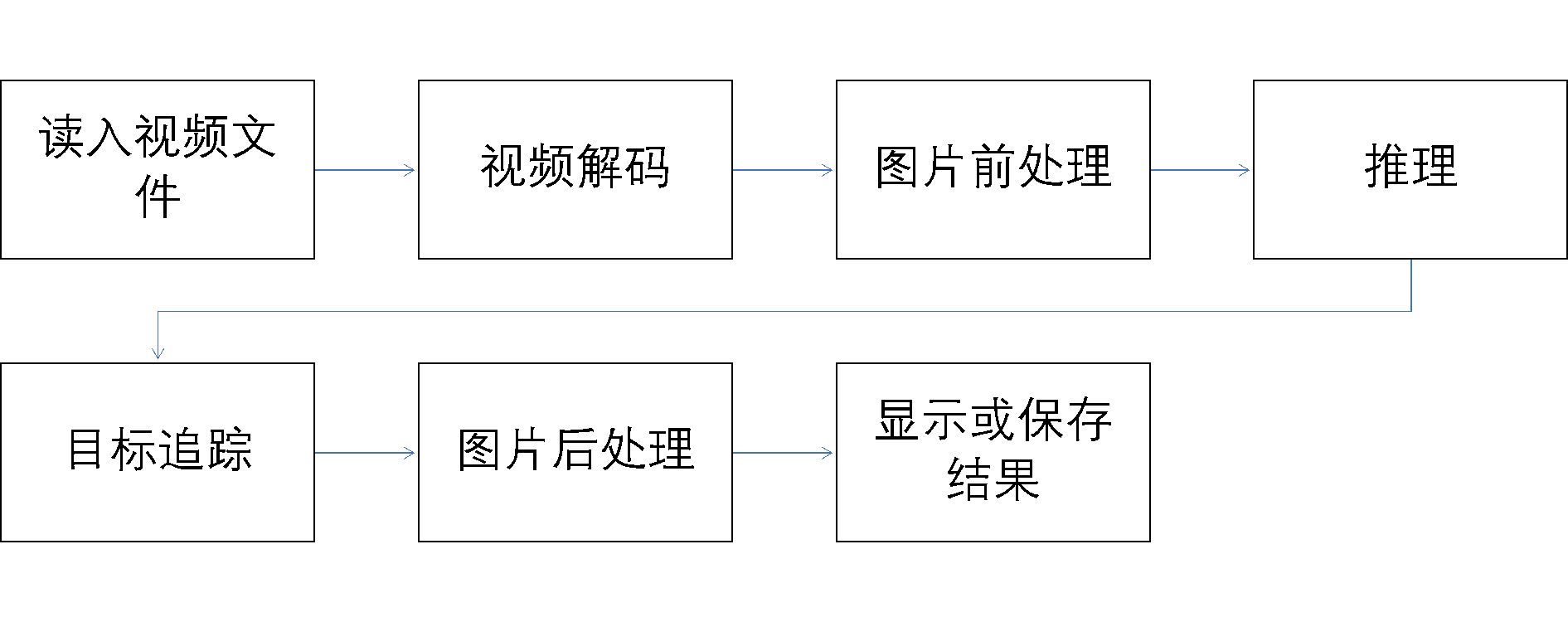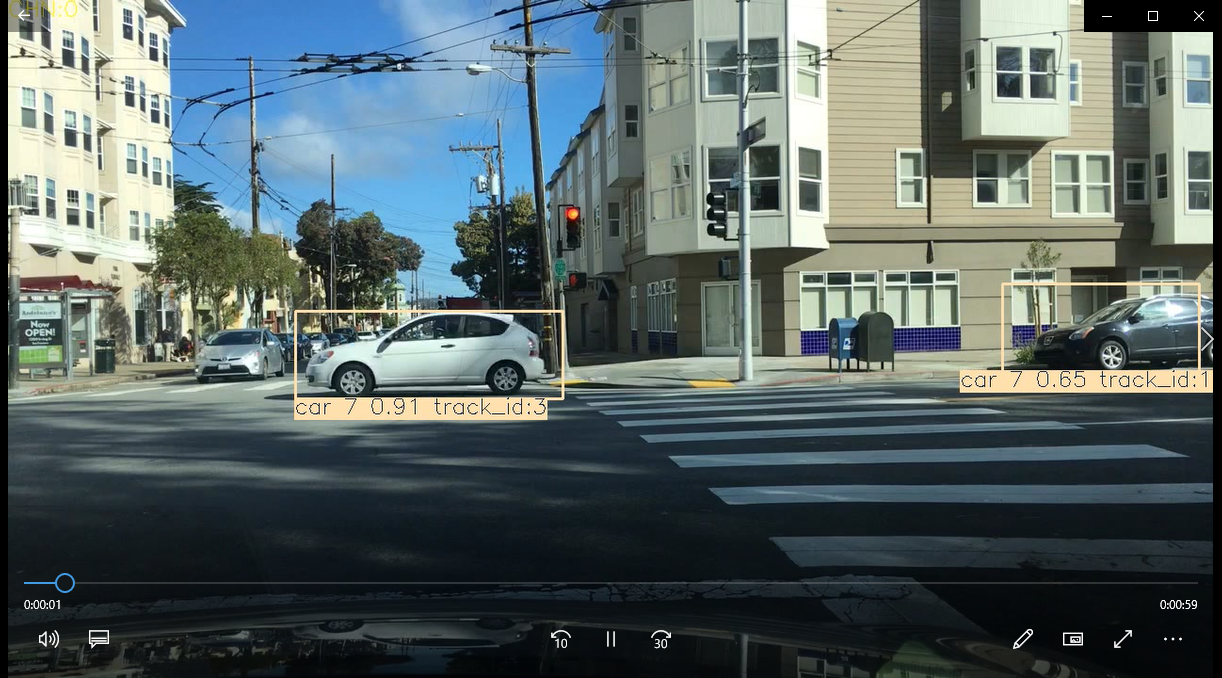### 一、源码获取与编译运行

`mkdir build`

`cd build/`

`cmake .. -DBUILD_SAMPLES=ON -DBUILD_TESTS=ON`

`make`

### 三、程序的具体运行流程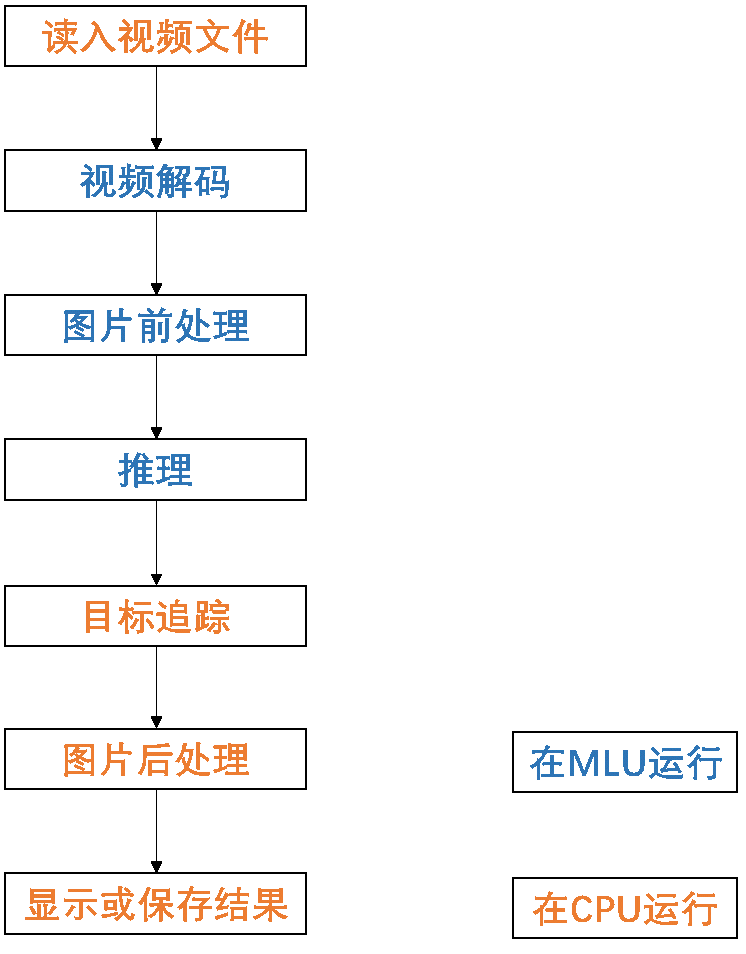MLU编程是一种异构编程，所以在程序运行过程中会涉及到数据流在Host 和 Device之间的相互拷贝。如上图所示，视频解码，图片前处理，推理这三步都在MLU上完成，所以这三步之间不需要设备和主机之间的内存拷贝。其中值得注意的是，在本例中，目标追踪的特征提取部分使用了openCV的特征提取API，也是在CPU上执行的。MLU上的特征提取和MLU 推理部分的原理类似，这里就不再赘述了。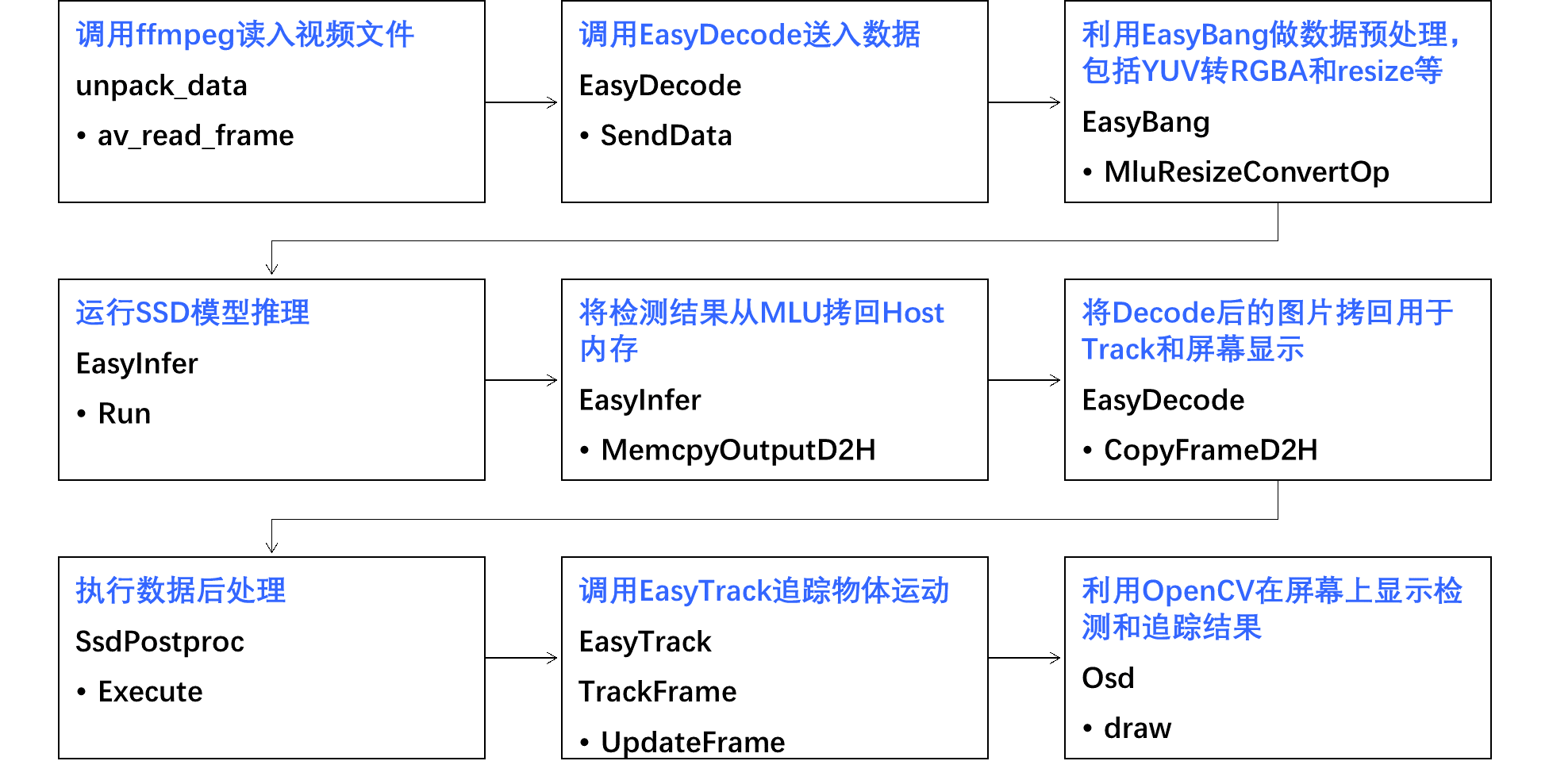### 四、MluContext的使用

`  edk::MluContext context;`

`    // set mlu environment`

`    context.SetDeviceId(0);`

`    context.BindDevice();`

### 五、EasyDecode的使用

EasyDecode的使用主要包括以下几个步骤。

1. 首先初始化解码器，利用edk::EasyDecode::Attr参数创建解码器实例。

`    edk::EasyDecode::Attr attr;`

`    attr.frame_geometry.w = 1920;`

`    attr.frame_geometry.h = 1080;`

`    attr.codec_type = edk::CodecType::H264;`

`    attr.pixel_format = edk::PixelFmt::NV21;`

`    attr.dev_id = 0;`

`    attr.frame_callback = decode_output_callback;`

`    attr.eos_callback = decode_eos_callback;`

`    attr.silent = false;`

`    attr.input_buffer_num = 6;`

`    attr.output_buffer_num = 6;`

`    decode = edk::EasyDecode::New(attr);`

`    g_decode = decode.get();`

2. 使用SendData将数据送入解码器。这里要注意解码器仅支持输入完整帧数据进行解码，建议使用 FFMpeg 进行解封装和 parse 后再送入解码器。FFMpeg相关的代码在unpack_data中。

`g_decode->SendData(pending_frame)`

3. 在解码完成之后可以将解码后的数据用于MLU推理或者拷回Host端做其他操作。具体的通过回调函数实现解码后的操作。

`void decode_output_callback(const edk::CnFrame &info) {`

`  std::unique_lock<std::mutex> lk(g_mut);`

`  g_frames.push(info);`

`  g_cond.notify_one();`

`}`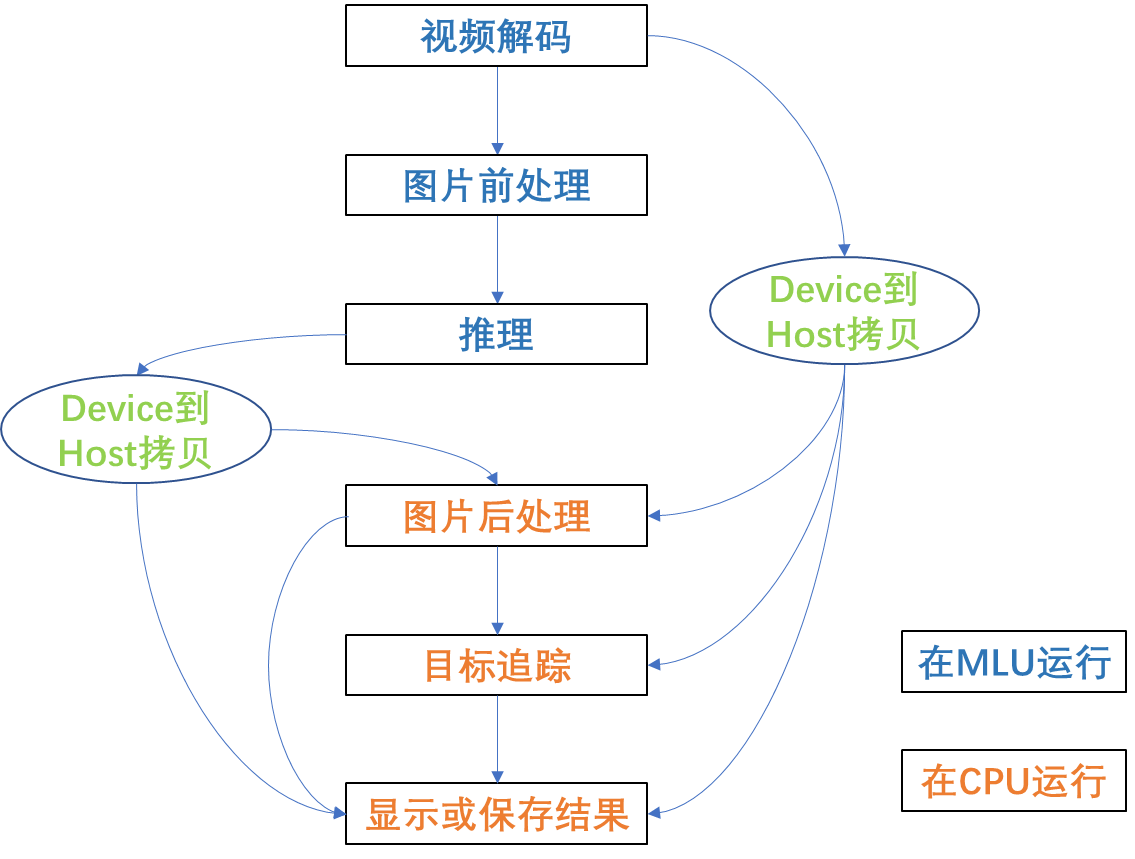` // copy out frame`

`      decode->CopyFrameD2H(img_data, frame);`

`      // release codec buffer`

`      decode->ReleaseBuffer(frame.buf_id);`

4. 最后一步是在整个视频数据发送完毕之后发送eos信息通知EasyDecode。EasyDecode的智能指针会在程序结束时自动析构并释放相关资源。

`void send_eos(edk::EasyDecode *decode) {`

`  edk::CnPacket pending_frame;`

`  pending_frame.data = nullptr;`

`  decode->SendData(pending_frame, true);`

`}`

### 六、EasyBang的使用

MluResizeConvertOp初始化部分的代码：

`      // Init resize and convert operator`

`      std::call_once(rcop_init_flag,`

`          [&] {`

`            // create mlu resize and convert op`

`            MluResizeConvertOp::Attr attr;`

`            attr.dst_h = in_shape.h;`

`            attr.dst_w = in_shape.w;`

`            attr.batch_size = 1;`

`            attr.core_version = context.GetCoreVersion();`

`            rc_op.SetMluQueue(infer.GetMluQueue());`

`            if (!rc_op.Init(attr)) {`

`              THROW_EXCEPTION(edk::Exception::INTERNAL, rc_op.GetLastError());`

`            }`

`          });`

`      // run resize and convert`

`      void *rc_output = mlu_input;`

`      edk::MluResizeConvertOp::InputData input;`

`      input.planes = frame.ptrs;`

`      input.planes = frame.ptrs;`

`      input.src_w = frame.width;`

`      input.src_h = frame.height;`

`      input.src_stride = frame.strides;`

`      rc_op.BatchingUp(input);`

`      if (!rc_op.SyncOneOutput(rc_output)) {`

`        g_running = false;`

`        g_exit = true;`

`        decode->ReleaseBuffer(frame.buf_id);`

`        THROW_EXCEPTION(edk::Exception::INTERNAL, rc_op.GetLastError());`

`      }`

### 七、EasyInfer的使用

`  std::shared_ptr<edk::ModelLoader> model;`

`  edk::MluMemoryOp mem_op;`

`  edk::EasyInfer infer;`

`    // load offline model`

`    model = std::make_shared<edk::ModelLoader>(FLAGS_model_path.c_str(), FLAGS_func_name.c_str());`

`    in_shape = model->InputShapes();`

`    out_shapes = model->OutputShapes();`

`    // prepare mlu memory operator and memory`

`    mem_op.SetModel(model);`

`    // init cninfer`

`    infer.Init(model, 0);`

`  void **mlu_input = mem_op.AllocMluInput();`

` ……`

`    mlu_output = mem_op.AllocMluOutput();`

`    cpu_output = mem_op.AllocCpuOutput();`

`      // run inference`

`      infer.Run(mlu_input, mlu_output);`

`      mem_op.MemcpyOutputD2H(cpu_output, mlu_output);`